# How to Calculate and Solve for Randomly Oriented Composite Modulus of Elasticity | Composites

The randomly oriented composite modulus of elasticity is illustrated by the image below.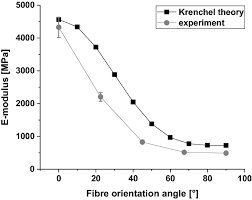To compute for randomly oriented composite modulus of elasticity, five essential parameters are needed and these parameters are Fibre Efficiency Parameter (K), Elastic Modulus of the Fibre (Ef), Elastic Modulus of the Matrix (Em), Volume Fraction of the Fibre (Vf) and Volume Fraction of the Matrix (Vm).

The formula for calculating randomly oriented composite modulus of elasticity:

E = KEfVf + EmVm

Where:

E = Randomly Oriented Composite Modulus of Elasticity
K = Fibre Efficiency Parameter
Ef = Elastic Modulus of the Fibre
Em = Elastic Modulus of the Matrix
Vf = Volume Fraction of the Fibre
Vm = Volume Fraction of the Matrix

Let’s solve an example;
Find the randomly oriented composite modulus of elasticity when the fibre efficiency parameter is 14, the elastic modulus of the fibre is 10, the elastic modulus of the matrix is 16, the volume fraction of the fibre is 12 and the volume fraction of the matrix is 8.

This implies that;

K = Fibre Efficiency Parameter = 14
Ef = Elastic Modulus of the Fibre = 10
Em = Elastic Modulus of the Matrix = 16
Vf = Volume Fraction of the Fibre = 12
Vm = Volume Fraction of the Matrix = 8

E = KEfVf + EmVm
E = (14)(10)(12) + (16)(8)
E = (1680) + (128)
E = 1808

Therefore, the randomly oriented composite modulus of elasticity is 1808 Pa.

Nickzom Calculator – The Calculator Encyclopedia is capable of calculating the randomly oriented composite modulus of elasticity.

To get the answer and workings of the randomly oriented composite modulus of elasticity using the Nickzom Calculator – The Calculator Encyclopedia. First, you need to obtain the app.

You can get this app via any of these means:

You can also try the demo version via https://www.nickzom.org/calculator

Apple (Paid) – https://itunes.apple.com/us/app/nickzom-calculator/id1331162702?mt=8
Once, you have obtained the calculator encyclopedia app, proceed to the Calculator Map, then click on Materials and Metallurgical under Engineering.Now, Click on Composites under Materials and MetallurgicalNow, Click on Randomly Oriented Composite Modulus of Elasticity under CompositesThe screenshot below displays the page or activity to enter your values, to get the answer for the randomly oriented composite modulus of elasticity according to the respective parameter which is the Fibre Efficiency Parameter (K), Elastic Modulus of the Fibre (Ef), Elastic Modulus of the Matrix (Em), Volume Fraction of the Fibre (Vf) and Volume Fraction of the Matrix (Vm).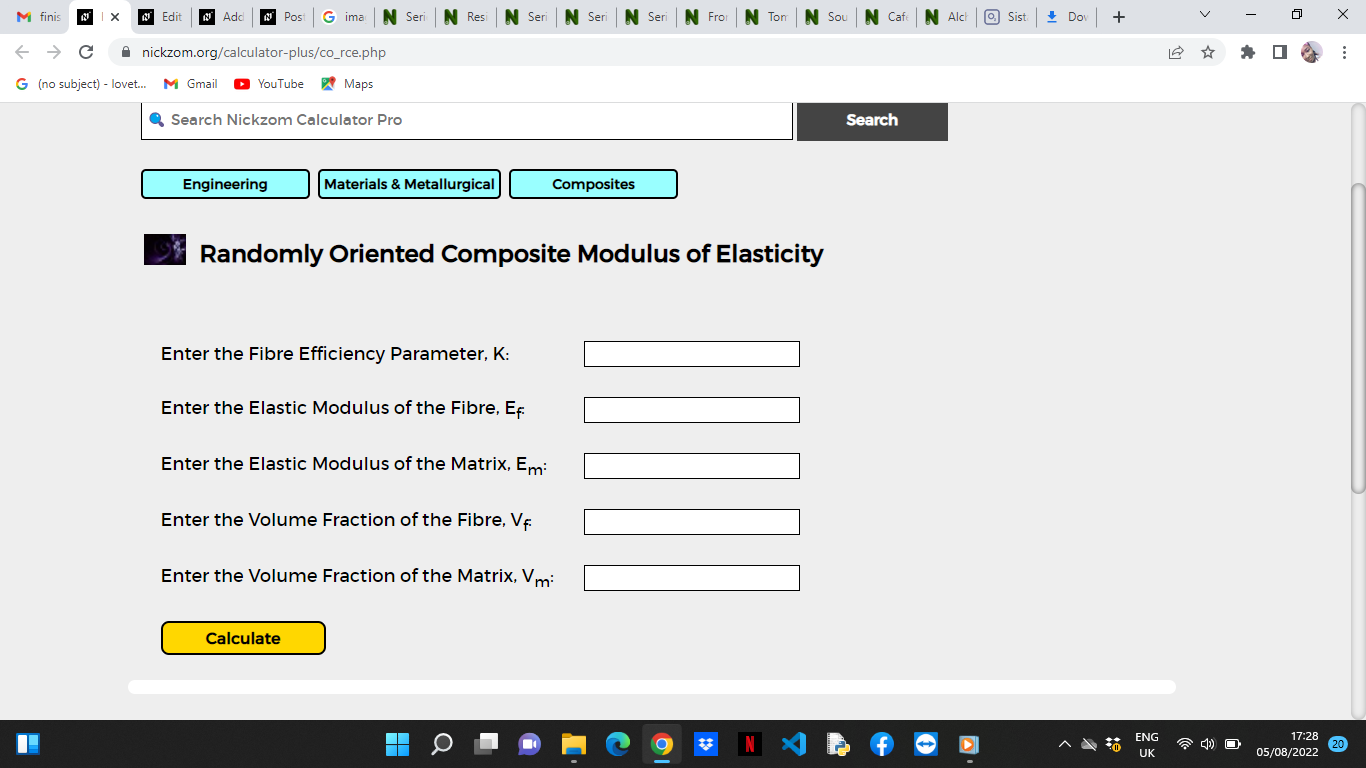Now, enter the values appropriately and accordingly for the parameters as required by the Fibre Efficiency Parameter (K) is 14, Elastic Modulus of the Fibre (Ef) is 10, Elastic Modulus of the Matrix (Em) is 16, Volume Fraction of the Fibre (Vfis 12 and Volume Fraction of the Matrix (Vm) is 8.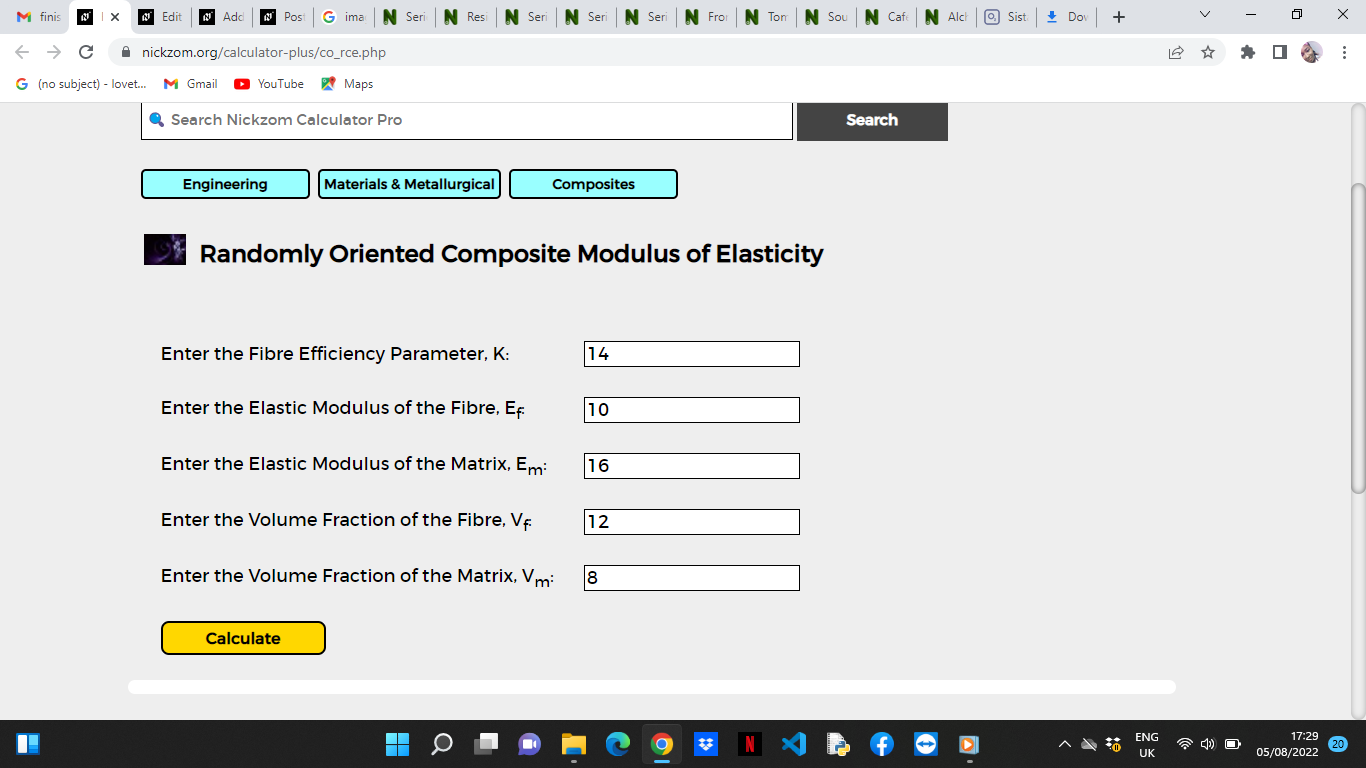Finally, Click on Calculate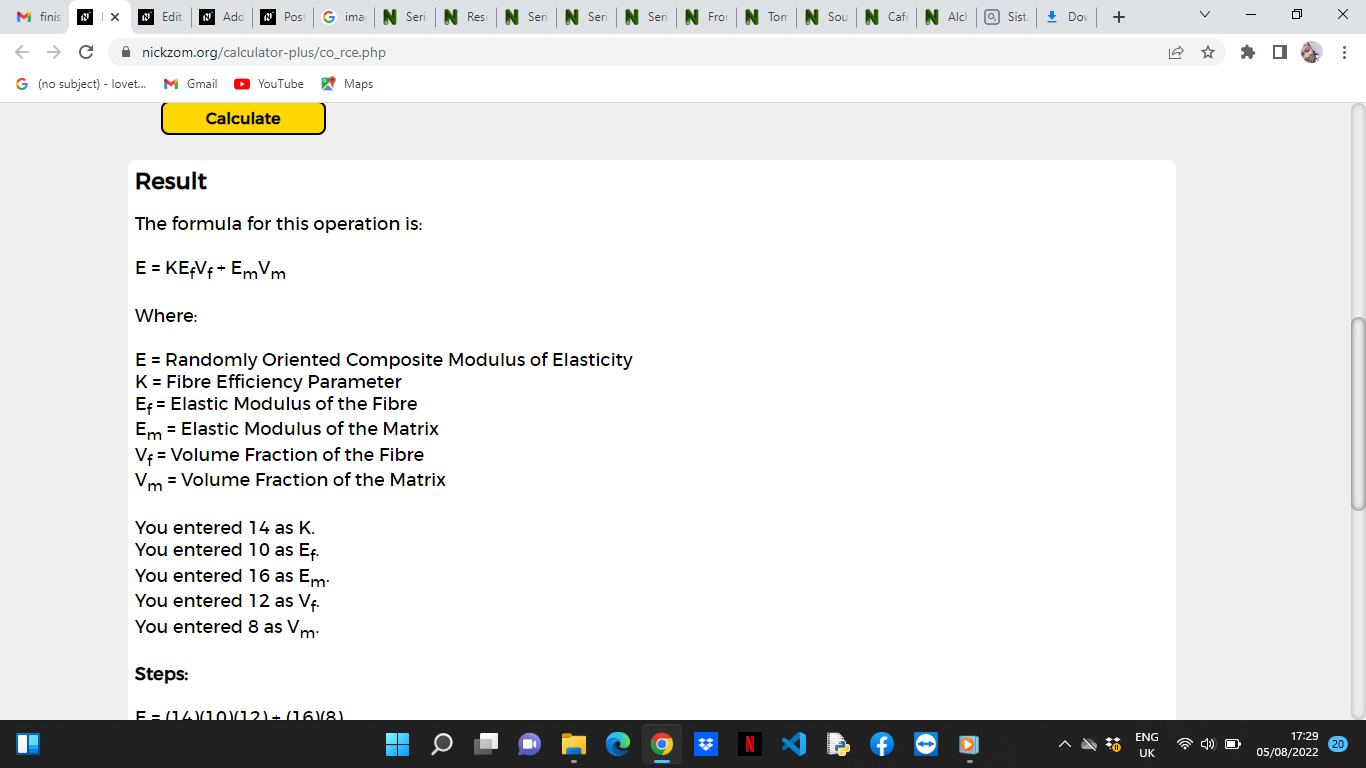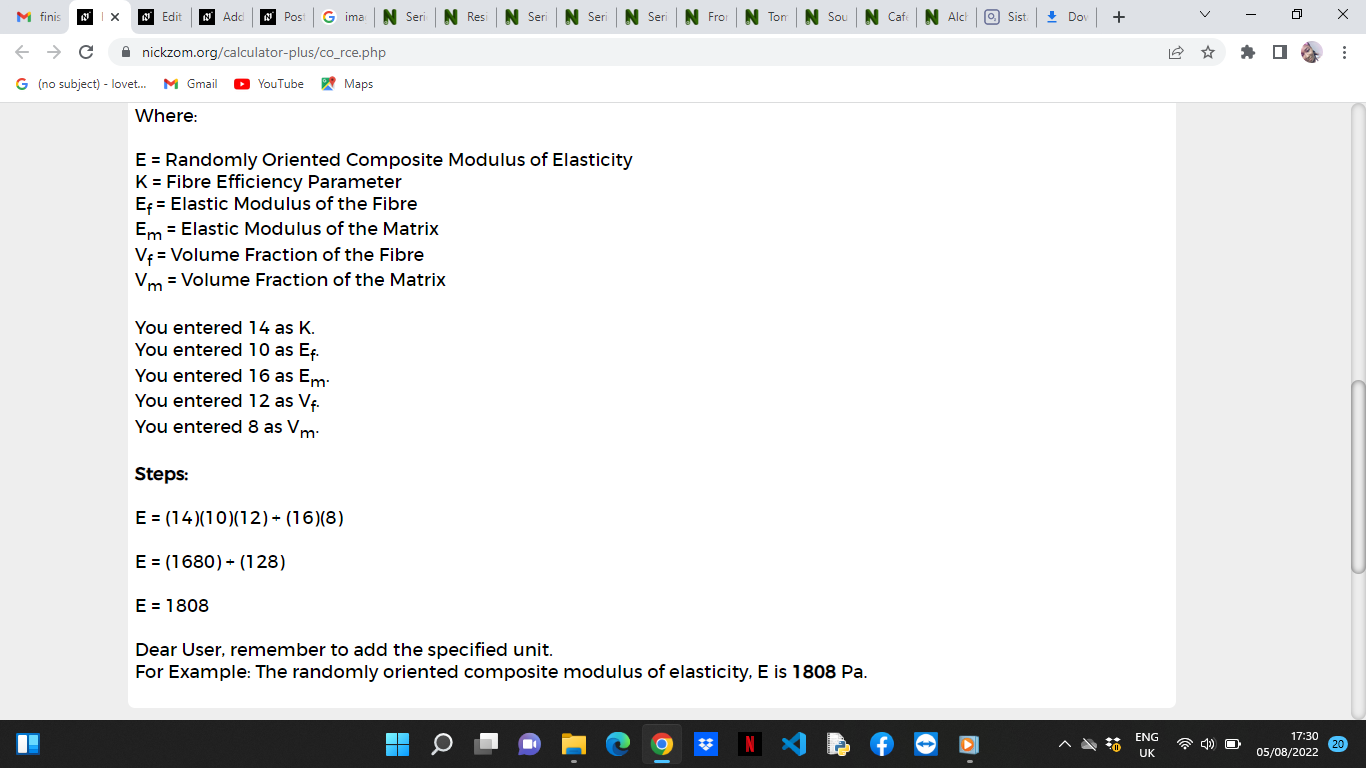As you can see from the screenshot above, Nickzom Calculator– The Calculator Encyclopedia solves for the randomoy oriented composite modulus of elasticity and presents the formula, workings and steps too.